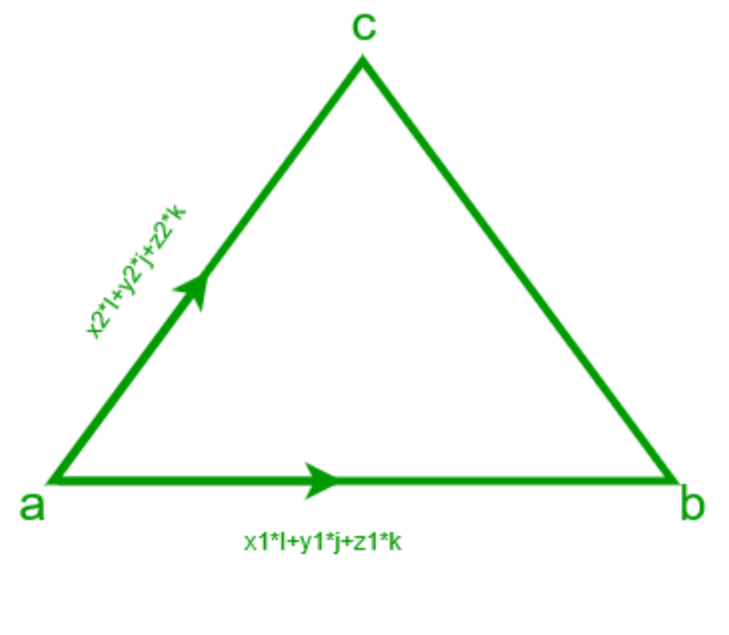# Find area of triangle if two vectors of two adjacent sides are given

Given two vectors in form of (xi+yj+zk) of two adjacent sides of a triangle. The task is to find out the area of a triangle.

Examples:

Input:
x1 = -2, y1 = 0, z1 = -5
x2 = 1, y2 = -2, z2 = -1
Output: Area = 6.422616289332565

Input:
x1 = -2, y1 = 1, z1 = 5
x2 = 1, y2 = 3, z2 = -1
Output: Area = 8.860022573334675

## Recommended: Please try your approach on {IDE} first, before moving on to the solution.Approach: Suppose we have two vectors a(x1*i+y1*j+z1*k) and b(x2*i+y2*j+z2*k) and we know that area of triangle is given by :

Area of triangle = (magnitude of cross product of vectors a and b) / 2 i.e |axb| / 2
And we know a X b = (y1*z2 – y2*z1)*i – (x1*z2 – x2*z1)*j + (x1*y2 – x2*y1)*k

Then area =## C++

 // C++ program to calculate area of    // triangle if vectors of   // 2 adjacent sides are given     #include  using namespace std ;     // function to calculate area of triangle    float area(int x1, int y1, int z1, int x2, int y2, int z2)  {      float area = sqrt(pow((y1 * z2 - y2 * z1),2)                + pow((x1 * z2 - x2 * z1),2) +                 pow((x1 * y2 - x2 * y1),2)) ;                  area = area / 2;      return area ;         }         // Driver Code   int main()  {      int    x1 = -2 ;      int y1 = 0 ;       int z1 = -5 ;      int x2 = 1 ;      int y2 = -2 ;      int z2 = -1 ;      float  a = area(x1, y1, z1, x2, y2, z2) ;        cout << "Area = " << a << endl;                                 return 0;  // This code is contributed by ANKITRAI1  }

## Java

 // Java program to calculate area of   // triangle if vectors of   // 2 adjacent sides are given  import java.util.*;     class solution  {  // function to calculate area of triangle   static float area(int x1, int y1, int z1, int x2, int y2, int z2)  {    double a =Math.pow((y1 * z2 - y2 * z1),2)               + Math.pow((x1 * z2 - x2 * z1),2) +                  Math.pow((x1 * y2 - x2 * y1),2);  float area = (float)Math.sqrt(a) ;             area = area / 2;      return area ;  }     //Driver program  public static void main(String arr[])  {      int x1 = -2 ;      int y1 = 0 ;       int z1 = -5 ;      int x2 = 1 ;      int y2 = -2 ;      int z2 = -1 ;      float a = area(x1, y1, z1, x2, y2, z2) ;       System.out.println("Area= "+a);  }     }  //This code is contributed by  //Surendra_Gangwar

## Python 3

 # Python code to calculate area of   # triangle if vectors of  # 2 adjacent sides are given  import math     # to calculate area of triangle   def area(x1, y1, z1, x2, y2, z2):      area = math.sqrt((y1 * z2 - y2 * z1) ** 2             + (x1 * z2 - x2 * z1) ** 2 +             (x1 * y2 - x2 * y1) ** 2)      area = area / 2     return area     # main function  def main():      x1 = -2     y1 = 0     z1 = -5     x2 = 1     y2 = -2     z2 = -1     a = area(x1, y1, z1, x2, y2, z2)      print("Area = ", a)     # driver code      if __name__=="__main__":      main()

## C#

 // C# program to calculate area    // of triangle if vectors of   // 2 adjacent sides are given  using System;  class GFG  {  // function to calculate area of triangle   static float area(int x1, int y1, int z1,                     int x2, int y2, int z2)  {  double a = Math.Pow((y1 * z2 - y2 * z1), 2) +              Math.Pow((x1 * z2 - x2 * z1), 2) +             Math.Pow((x1 * y2 - x2 * y1), 2);  float area = (float)Math.Sqrt(a) ;             area = area / 2;      return area ;  }     // Driver Code  public static void Main()  {      int x1 = -2;      int y1 = 0;       int z1 = -5;      int x2 = 1;      int y2 = -2;      int z2 = -1;      float a = area(x1, y1, z1, x2, y2, z2);       Console.WriteLine("Area = " + a);  }  }     // This code is contributed  // by inder_verma

## PHP

 

Output:

Area =  6.422616289332565


My Personal Notes arrow_drop_upCheck out this Author's contributed articles.

If you like GeeksforGeeks and would like to contribute, you can also write an article using contribute.geeksforgeeks.org or mail your article to contribute@geeksforgeeks.org. See your article appearing on the GeeksforGeeks main page and help other Geeks.

Please Improve this article if you find anything incorrect by clicking on the "Improve Article" button below.